# Exciting Maths Worksheets for KidsPresenting you the best-in-class Maths Worksheets for kids that encompasses various arithmetic operations- addition, multiplication, division, word problems and subtraction worksheets for kindergarten. It also includes counting worksheets for kindergarten. These worksheets for kids enhance your child’s mental power and boost his/her confidence to solve simple Maths addition sums easily.

Given below are some of the most exciting Maths Worksheets for kids which your kids would love to solve. Why wait? Let’s explore! Just click on the images given below to download the colourful PDFs for free and let your child have a fun-filled learning experience.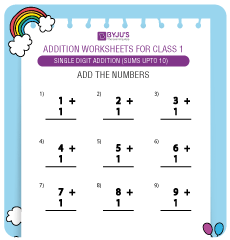##### Single Digit Addition Worksheet 1 (Sums up to 10)

Here is an exciting worksheet for kids on single-digit addition (Sums up to 10).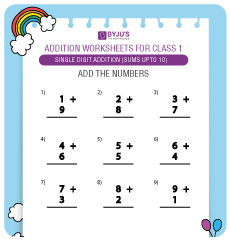##### Single Digit Addition Worksheet 2 (Sums up to 10)

Solve this worksheet on single-digit addition, where the sum of the numbers will be up to 10.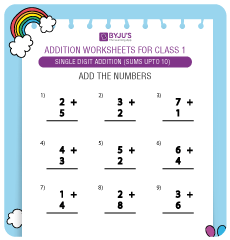##### Single Digit Addition Worksheet 3 (Sums up to 10)

Open and solve this worksheet on single-digit addition, the sum of numbers will be up to 10.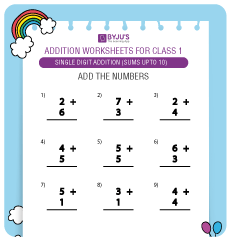##### Single Digit Addition Worksheet 4 (Sums up to 10)

Explore this interesting worksheet on single-digit addition (Sums up to 10).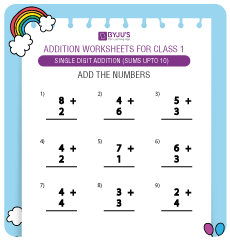##### Single Digit Addition Worksheet 5 (Sums up to 10)

Practice this amazing worksheet on single-digit addition (Sums up to 10).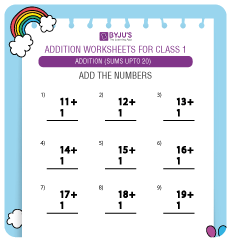##### Single Digit Addition Worksheet 1 (Sums up to 20)

Open this worksheet on single-digit addition for sums up to 20.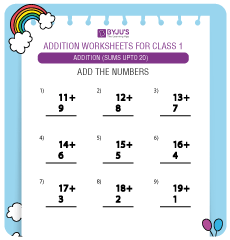##### Single Digit Addition Worksheet 2 (Sums up to 20)

Practice from an interesting worksheet about single-digit addition for the sums of numbers up to 20.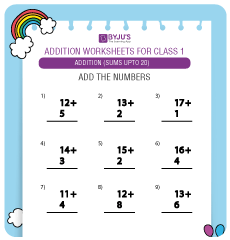##### Single Digit Addition Worksheet 3 (Sums up to 20)

Explore the worksheet for an amazing set of single-digit addition questions for simple addition sums up to 20.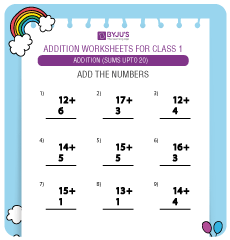##### Single Digit Addition Worksheet 4 (Sums up to 20)

Here’s an interesting collection of single-digit addition problems in the worksheet for sums up to 20 for practice.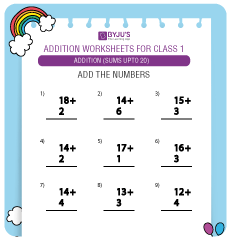##### Single Digit Addition Worksheet 5 (Sums up to 20)

Check this worksheet to solve single-digit addition questions where the sums of numbers is up to 20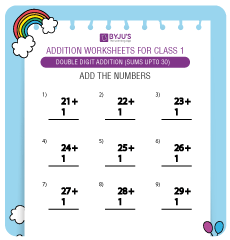##### Double Digit Addition Worksheet 1

Here’s an interesting collection of double-digit addition problems in the worksheet for sums up to 30 for practice.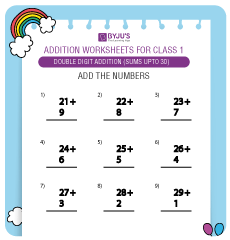##### Double Digit Addition Worksheet 2

Explore the worksheet for an amazing set of double-digit addition questions for simple addition sums up to 30.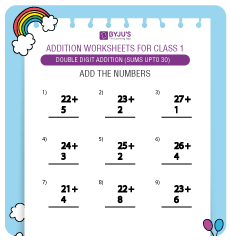##### Double Digit Addition Worksheet 3

Open this activity sheet on double-digit addition for sums up to 30.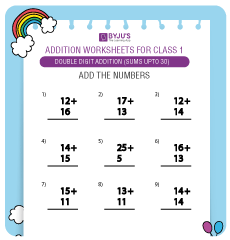##### Double Digit Addition Worksheet 4

Check this worksheet to solve double-digit addition questions where the sums of numbers is up to 30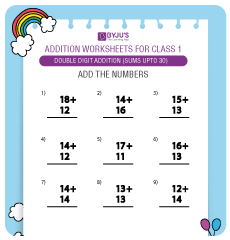##### Double Digit Addition Worksheet 5

Practice from an interesting worksheet about double-digit addition for the sums of numbers up to 30.

### Other Resources for Kids:

We definitely hope your children had an amazing experience solving the above Maths Worksheets for kids. Here are some more worksheets on different subjects that your kids would love to sol

Enjoyed solving the kids activity sheets of simple Maths addition sums? You can access more such interesting resources in our Kids Learning section such as poems, essays, stories, GK Questions, NCERT solutions, trivia questions, etc. and give your child joyful and prosperous learning experience. Keep an eye on BYJU’S website to learn more.